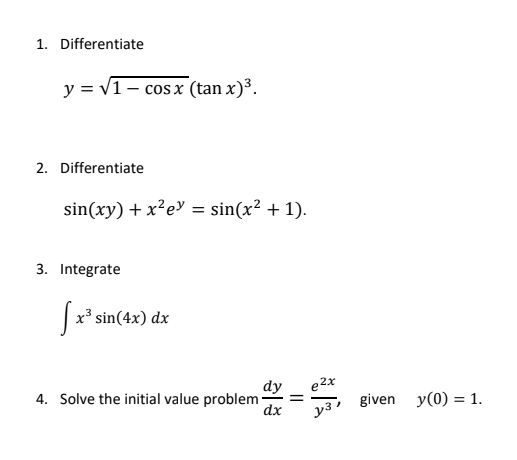### Create an Account

Home / Questions / 1. Differentiate y = V1 - cos x (tan x)3. 2. Differentiate sin(xy) + xạey = sin(x2 + 1). 3...

# 1. Differentiate y = V1 - cos x (tan x)3. 2. Differentiate sin(xy) + xạey = sin(x2 + 1). 3. Integrate ſ r* sin(4x) dx 4. Solve the initial value problem dy dx e27 331 given y(0) = 1.

1. Differentiate y = V1 - cos x (tan x)3. 2. Differentiate sin(xy) + xạey = sin(x2 + 1). 3. Integrate ſ r* sin(4x) dx 4. Solve the initial value problem dy dx e27 331 given y(0) = 1.May 09 2021 View more View LessSubscribe To Get Solution